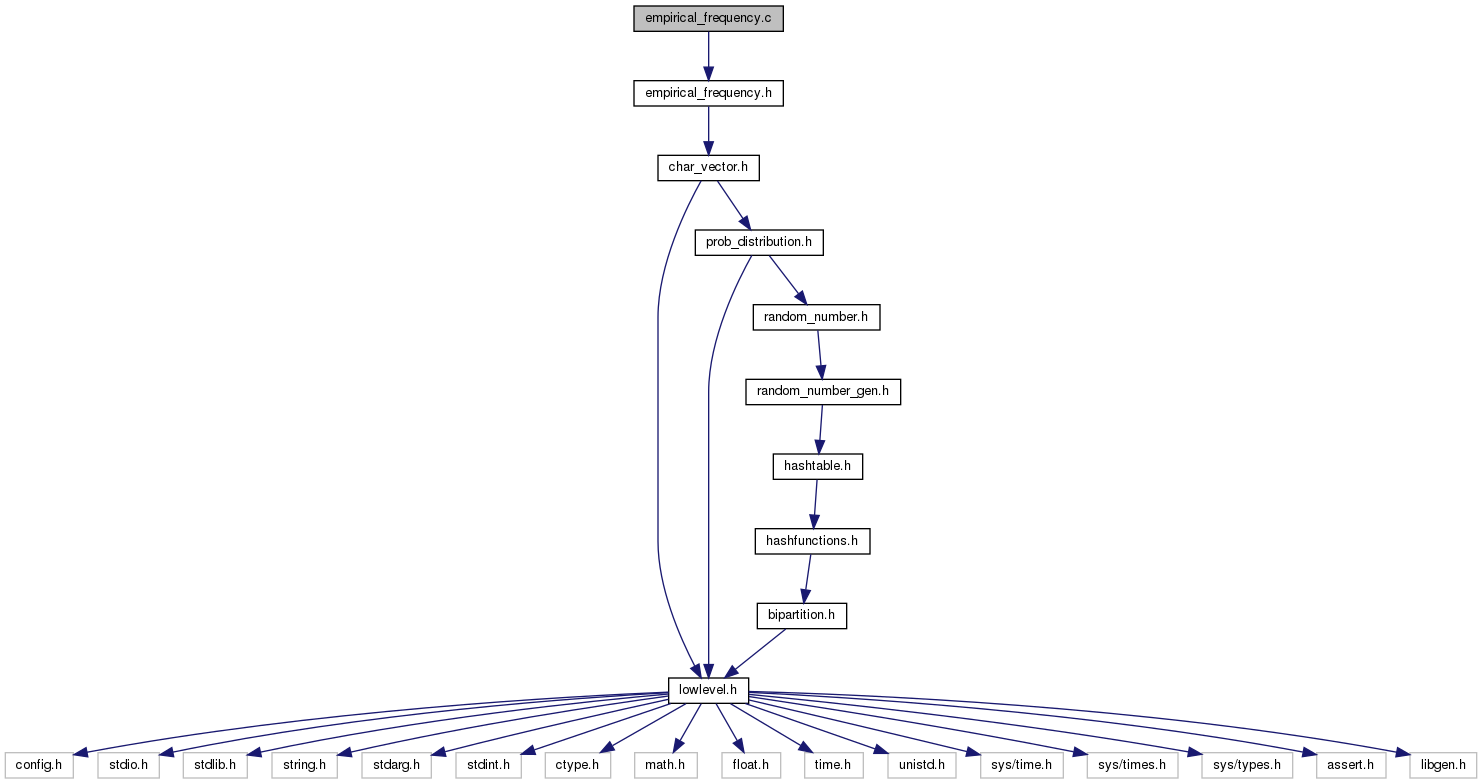biomcmc-lib  0.1 low level library for phylogenetic analysis
empirical_frequency.c File Reference

histogram of vectors, ordered by frequency. Also calculates MAP (modal) values. More...

`#include "empirical_frequency.h"`
Include dependency graph for empirical_frequency.c:## Functions

int compare_empfreq_element_decreasing (const void *a, const void *b)

int compare_empfreq_element_increasing (const void *a, const void *b)

int compare_empfreq_double_element_decreasing (const void *a, const void *b)

int compare_empfreq_double_element_increasing (const void *a, const void *b)

empfreq create_empfreq_from_value_sorted_empfreq (empfreq e_idx)

void sort_empfreq_decreasing (empfreq ef)

void sort_empfreq_increasing (empfreq ef)

void sort_empfreq_double_decreasing (empfreq_double efd)

void sort_empfreq_double_increasing (empfreq_double efd)

empfreq new_empfreq (int n_elements)

void del_empfreq (empfreq ef)

empfreq_double new_empfreq_double (int n_elements)

void del_empfreq_double (empfreq_double efd)

empfreq new_empfreq_sort_decreasing (void *vec, int n, char type)

empfreq new_empfreq_sort_increasing (void *vec, int n, char type)

empfreq_double new_empfreq_double_sort_decreasing (double *vec, int n)

empfreq_double new_empfreq_double_sort_increasing (double *vec, int n)

empfreq new_empfreq_from_int (int *vec, int n)

empfreq new_empfreq_from_int_weighted (int *vec, int n, int *weight)

int find_mode_int (int *vec, int n)

int find_mode_int_weighted (int *vec, int n, int *weight)

## Detailed Description

histogram of vectors, ordered by frequency. Also calculates MAP (modal) values.

Sorts a vector of integers by their frequencies, preserving their original indexes. It is a simple extension to qsort where the original order can be reconstructed, or still a key/value sorting.